# Homeschooling With The Math Translator

The Math Translator courses provide comprehensive and high-quality video instruction in math from a professor with 20+ years of teaching experience and a real gift and passion for teaching math. The courses utilize the free online OpenStax™ math textbooks.  The textbooks can be accessed directly from each lesson page.  Each course contains comprehensive video lessons for each section of the corresponding book, homework support videos, and answer keys for the practice tests in each book.

## Why should I homeschool with The Math Translator?

The most common platforms for online instruction these days are Learning Management Systems (LMS). A LMS is a software program that provides online homework and walks students through homework problems if they struggle with a problem.  These platforms are good in theory, but from my years of teaching in the online format I have learned that LMS programs don't actually translate into success for the student. I have found two main issues with the LMS programs that decrease their efficacy.

The first problem is that LMS programs generally do not provide comprehensive instruction.   If they provide videos, they are generally short "snippet" videos. Snippet videos do not provide enough background for a student to fully comprehend the concepts.  There is a reason that class periods are an hour long! The second issue I have found with LMS programs is that the act of doing homework on a computer is fundamentally different than doing homework on paper.  There is something about writing on paper that is crucial for the process of learning mathematics.

##### The Math Translator provides a high-quality alternative to LMS programs

A few years ago I removed all of my online classes from LMS programs and instead recreated the face to face environment in the online setting and my success rates soared!  The traditional method of learning mathematics really is the best format for learning the subject.  That format involves comprehensive instruction from a qualified instructor, the student actively participating in the instruction by taking notes, the student applying what they learn by doing homework by hand with a pencil and paper out of a book and not on a computer program, and then assessments that test what the student has learned.  The Math Translator courses are structured to provide all of these elements in a straight forward and user friendly model that provides a much needed alternative to the LMS programs currently available on the market.

## How can I use The Math Translator courses to homeschool my child in math?

The Math Translator courses are best used as a direct replacement for the instruction received at a brick and mortar school.  Enrollment can happen at anytime and proceed at any pace that is comfortable for the student.  Take a few moments to listen to Melissa speak about how The Math Translator courses work.

• #### Step 1

Students watch the video lessons and take notes as they would if they were in class in person.

• #### Step 2

Students do the suggested homework exercises listed on each lesson page. The homework is done out of the free online OpenStax™ math textbooks. Students can check their answers on the homework problems in the OpenStax book and if they have questions they can reference the homework support videos posted on each lesson page.

• #### Step 3

At the end of each chapter, students study for the test and take the Practice Test provided in the OpenStax textbook.  Students, or parents, can then grade the test using the "Practice Test Answer Keys" posted on the homepage of the video library.

## Orientation Video

If you wish to have a more detailed look at how The Math Translator courses work beyond what is contained in the "How It Works" video above, please feel free to watch the full orientation video below.

The Math Translator courses take you back to the basics because learning math does not have to be complicated.  Each course is composed of three elements: comprehensive video lectures, homework support videos, and written answer keys for each practice test in the book.  The course outlines give an overview of the content of each lecture as well as suggested homework exercises.  The course calendars give a suggested timeline to complete each course within a 9-month period. The answer keys for the Chapter 1 Practice Tests have been posted here; for the rest of the answer keys, please login.

•#### Prealgebra

Recommended for grades 7 or 8 (approximately 12 years old)

This course covers the fundamental arithmetic and geometry skills needed for success in algebra.  Topics include whole numbers, the language of algebra, integers, fractions, decimals, percents, the properties of real numbers, solving linear equations, math models and geometry, polynomials, and graphs. This course contains 62 comprehensive 1-hour lectures and 963 homework support videos, in addition to answer keys for each practice test in the book.

Course Outline

Course Calendar

Chapter 1 - Practice Test Answer Key.pdf

•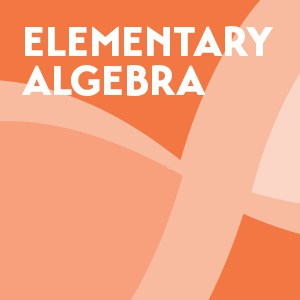#### Elementary Algebra (Algebra I)

Recommended for grades 8 or 9 (approximately 13 - 14 years old)

This course covers the fundamental concepts of algebra.  Topics include solving linear equations and inequalities, math models, linear graphs, systems of linear equations, polynomials, factoring, rational expressions and equations, roots and radicals, and quadratic equations. This course contains 80 comprehensive 1-hour lectures and 1,116 homework support videos, in addition to answer keys for each practice test in the book.

Recommended Prerequisite: Prealgebra

Course Outline

Course Calendar

Chapter 1 - Practice Test Answer Key.pdf

•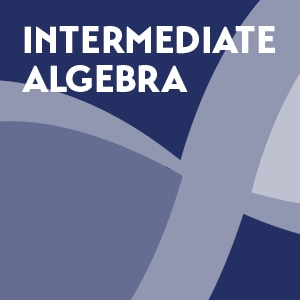#### Intermediate Algebra (Algebra II)

Recommended for grades 10 or 11 (approximately 15 - 16 years old)

This course covers algebra concepts in the depth needed to prepare students for college algebra.  Topics include solving linear equations, graphs and functions, systems of linear equations, polynomials and polynomial functions, factoring, rational expressions and functions, roots and radicals, quadratic equations and functions, exponential and logarithmic functions, conic sections, and series, sequences, and the binomial theorem. This course contains 95 comprehensive 1-hour lectures and 1,021 homework support videos, in addition to answer keys for each practice test in the book.

Recommended Prerequisite: Elementary Algebra

Course Outline

Course Calendar

Chapter 1 - Practice Test Answer Key.pdf

•#### College Algebra

Recommended for grades 11 and/or 12 for students not planning on majoring in math or science (approximately 16 - 18 years old)

This course provides a comprehensive exploration of algebra principles. Topics include equations and inequalities, functions, linear functions, polynomial and rational functions, exponential and logarithmic functions, systems of equations and inequalities, analytic geometry, sequences, probability, and counting theory. This course contains 65 comprehensive 1-hour lectures and 730 homework support videos, in addition to answer keys for each practice test in the book.

Recommended Prerequisite: Intermediate Algebra

Course Outline

Course Calendar

Chapter 1 - Practice Test Answer Key.pdf

•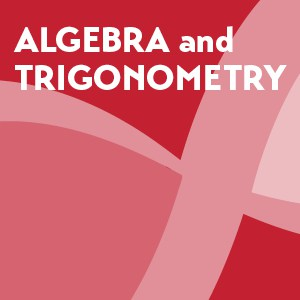#### Algebra and Trigonometry

Recommended for grades 11 and/or 12 for students that are uncertain of whether they will major in math or science (approximately 16 - 18 years old)

This course provides in depth coverage of algebra and trigonometry principles. Topics include equations and inequalities, functions, linear functions, polynomial and rational functions, exponential and logarithmic functions, the unit circle, periodic functions, trigonometric identities and equations, further applications of trigonometry, systems of equations and inequalities, analytic geometry, sequences, probability, and counting theory. This course contains 85 comprehensive 1-hour lectures and 974 homework support videos, in addition to answer keys for each practice test in the book.

Recommended Prerequisite: Intermediate Algebra

Course Outline

Course Calendar

Chapter 1 - Practice Test Answer Key.pdf

•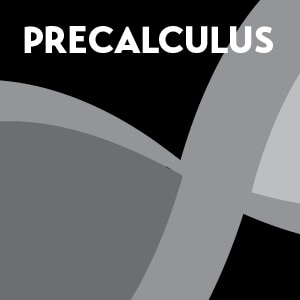#### Precalculus

Recommended for grades 11 and/or 12 for students that are certain they will major in math or science (approximately 16 - 18 years old)

This course covers the algebra and trigonometry principles needed for success in calculus. Topics include functions, linear functions, polynomial and rational functions, exponential and logarithmic functions, trigonometric functions, periodic functions, trigonometric identities and equations, further applications of trigonometry, systems of equations and inequalities, analytic geometry, and sequences, probability, and counting theory. This course contains 73 comprehensive 1-hour lectures and 800 homework support videos, in addition to answer keys for each practice test in the book.

Recommended Prerequisite: Intermediate Algebra

Course Outline

Course Calendar

Chapter 1 - Practice Test Answer Key.pdf

## How do I know which course to place my child in?

Proper placement is important for your student's success in these courses. Typically students will progress through math in this order: Prealgebra > Elementary Algebra (Algebra I) > Intermediate Algebra (Algebra II) > One of these courses: College Algebra, Algebra and Trigonometry, or Precalculus (please see the flow diagram below for more information on this). The two areas of entry into The Math Transaltor courses where there is typically some uncertainty as to which course to take is at the juncture between Prealgebra and Elementary Algebra, and Intermediate Algebra and one of the college level courses.  Please use the following placement exams to decide placement in those courses.  If you are still uncertain as to where to place your child, feel free to contact me for assistance in the process.

•#### Placement Test - Level I

Use this placement test to decide between the Prealgebra and Elementary Algebra courses.

Placement Test - Level I.pdf

Placement Test - Level I - Answer Key.pdf

•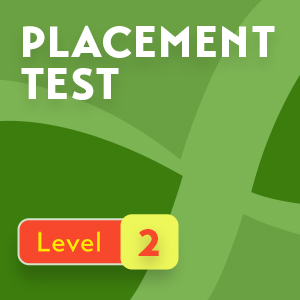#### Placement Test - Level II

Use this placement test to decide between the Elementary Algebra and Intermediate Algebra courses.

Placement Test - Level II.pdf

Placement Test - Level II - Answer Key.pdf

•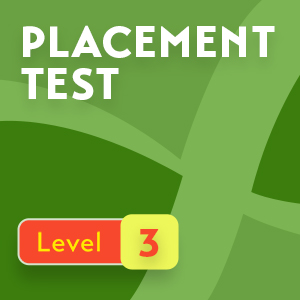#### Placement Test - Level III

Use this placement test to decide between Intermediate Algebra and one of the following courses: College Algebra, Algebra and Trigonometry, or Precalculus.

Placement Test - Level III.pdf

Placement Test - Level III - Answer Key.pdf

## What sequence of classes should my child follow?

Below is a flow diagram that depicts the best order in which to complete The Math Translator courses.

•••#### Next choose one of the following courses based on student interest in mathematics and intended major in college

•#### For students not intending to major in math or science in college

•#### For students that are uncertain if they will major in math or science in college

•### Testimonials

"Melissa is the best math teacher I have ever had!"

"Melissa has helped me understand concepts that I would have struggled with before."

"I've learned more from Melissa than any other instructor."

"Melissa has taught me how to think outside the box, especially with word problems."

"Melissa is really great at explaining concepts."